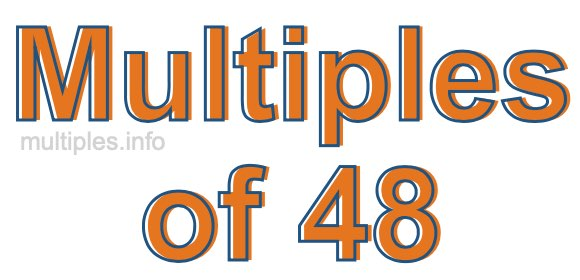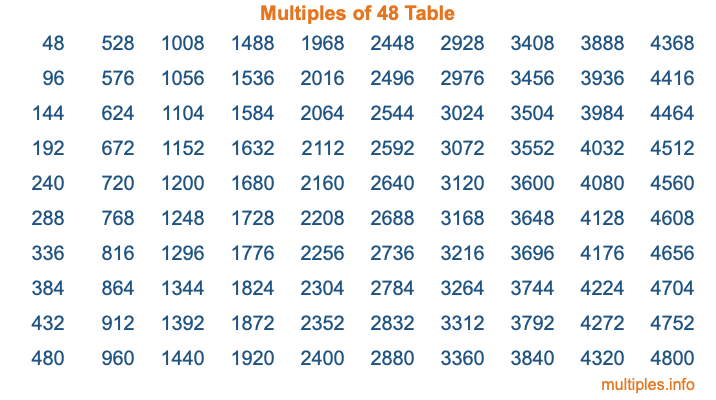Multiples of 48Welcome to the Multiples of 48 page. Here we will first teach you everything you will ever need to know about the multiples of 48, and then give you a study guide summary of everything we taught you to make sure you remember it all. Use this page to look up facts and learn information about the multiples of 48. This page will make you a multiples of forty-eight expert!

Definition of Multiples of 48
Multiples of 48 are all the numbers that when divided by 48 equal an integer. Each of the multiples of 48 are called a multiple. A multiple of 48 is created by multiplying 48 by an integer.

Therefore, to create a list of multiples of 48, you start with 1 multiplied by 48, then 2 multiplied by 48, then 3 multiplied by 48, and so on for as long as you want. Thus, the list of the first five multiples of 48 is 48, 96, 144, 192, and 240. To see a larger list of multiples of 48, see the printable image of Multiples of 48 further down on this page. We also have a category where you can choose any nth multiple of 48.

Multiples of 48 Checker
The Multiples of 48 Checker below checks to see if any number of your choice is a multiple of 48. In other words, it checks to see if there is any number (integer) that when multiplied by 48 will equal your number. To do that, we divide your number by 48. If the the quotient is an integer, then your number is a multiple of 48.

Is  a multiple of 48?

Least Common Multiple of 48 and ...
A Least Common Multiple (LCM) is the lowest multiple that two or more numbers have in common. This is also called the smallest common multiple or lowest common multiple and is useful to know when you are adding our subtracting fractions. Enter one or more numbers below (48 is already entered) to find the LCM.

Check out our LCM Calculator if you need more details about the Least Common Multiple or if you need the LCM for different numbers for adding and subtraction fractions.

nth Multiple of 48
As we stated above, 48 is the first multiple of 48, 96 is the second multiple of 48, 144 is the third multiple of 48, and so on. Enter a number below to find the nth multiple of 48.

th multiple of 48

Multiples of 48 vs Factors of 48
48 is a multiple of 48 and a factor of 48, but that is where the similarities end. All postive multiples of 48 are 48 or greater than 48. All positive factors of 48 are 48 or less than 48.

Below is the beginning list of multiples of 48 and the factors of 48 so you can compare:

Multiples of 48: 48, 96, 144, 192, 240, etc.

Factors of 48: 1, 2, 3, 4, 6, 8, 12, 16, 24, 48

As you can see, the multiples of 48 are all the numbers that you can divide by 48 to get a whole number. The factors of 48, on the other hand, are all the whole numbers that you can multiply by another whole number to get 48.

It's also interesting to note that if a number (x) is a factor of 48, then 48 will also be a multiple of that number (x).

Multiples of 48 vs Divisors of 48
The divisors of 48 are all the integers that 48 can be divided by evenly. Below is a list of the divisors of 48.

Divisors of 48: 1, 2, 3, 4, 6, 8, 12, 16, 24, 48

The interesting thing to note here is that if you take any multiple of 48 and divide it by a divisor of 48, you will see that the quotient is an integer.

Multiples of 48 Table
Below is an image of the first 100 multiples of 48 in a table. The table is in chronological order, column by column. The first column has the first ten multiples of 48, the second column has the next ten multiples of 48, and so on.The Multiples of 48 Table is also referred to as the 48 Times Table or Times Table of 48. You are welcome to print out our table for your studies.

Negative Multiples of 48
Although not often discussed or needed in math, it is worth mentioning that you can make a list of negative multiples of 48 by multiplying 48 by -1, then by -2, then by -3, and so on, to get the following list of negative multiples of 48:

-48, -96, -144, -192, -240, etc.

Multiples of 48 Summary
Below is a summary of important Multiples of 48 facts that we have discussed on this page. To retain the knowledge on this page, we recommend that you read through the summary and explain to yourself or a study partner why they hold true.

There are an infinite number of multiples of 48.

A multiple of 48 divided by 48 will equal a whole number.

48 divided by a factor of 48 equals a divisor of 48.

The nth multiple of 48 is n times 48.

The largest factor of 48 is equal to the first positive multiple of 48.

48 is a multiple of every factor of 48.

48 is a multiple of 48.

A multiple of 48 divided by a divisor of 48 equals an integer.

48 divided by a divisor of 48 equals a factor of 48.

Any integer times 48 will equal a multiple of 48.

Multiples of a Number
Here you can get the multiples of another number, all with the same attention to detail as we did for multiples of 48 on this page.

Multiples of
Multiples of 49
Did you find our page about multiples of forty-eight educational? Do you want more knowledge? Check out the multiples of the next number on our list!